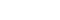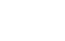Investing Guides

## Gross Rental Yield

Gross Rental Yield is the annual rental income before any expenses, divided by purchase price. Gives you a very general idea of the investment worth as well as a quick and easy way to compare different properties to each other – as long as the costs associated with each property are likely to be similar. While the gross yield does not take into account costs as well as the time value of money, it is still a useful screening tool used by investors when evaluating potential investments. Dubai’s average gross yield ranges between 7% and 11% which are amongst the highest in the world. A good gross yield isn’t always the same as a high gross yield. For example, as gross yield doesn't factor in the expenses associated with the upkeep of a property, high maintenance costs or service charges might bring down the profit substantially. Sometimes, rental property with the highest yields may also have more risk or a lower level of appreciation. However, a high gross yield also means there is more gross cash flow coming in. A mistake many beginners to real estate investments make is to focus on only one criteria when analysing rental property performance. While gross yield is important, three other rental yield calculations should be considered in addition to gross yield: Net yield, cap rate, and cash-on-cash return.

## Net Rental Yield

Net Rental Yield is the annual net profit (rental income minus costs), divided by purchase price. Costs may include loan payments, management fees, insurance, repairs, voids, service charge, etc. This makes the net yield more accurate than gross yield as it's based on the actual amount of money you'll end up with after costs. It tells you whether investing in a certain property given its particular expenses is a wise move or not. While the net yield does not take into account the time value of money, it’s still a useful screening tool used by investors when evaluating potential investments. Dubai’s average net yield ranges between 5% and 9% which are amongst the highest in the world. A rental yield of this figure ensures the investor is still making a significant return on their investment, even after loan payments, expenses, letting void periods, and more. Many investors consider rental yields as their go-to metric when buying an investment property. People invest in real estate to enjoy a cash flow of rental returns. Ensuring that you generate a high rental return is crucial if you want to maximise income. However, while important, rental yields aren’t the be-all and end-all of a buy to let investment. The best buy to let investment strategies combine a good rental yield with capital appreciation and tenant demand. Say, for instance, you buy a property with an average rental yield of 8%. A high average rental yield is great, but imagine that the neighbourhood / building the property is based in shows no sign of future property price growth. The property itself also struggles to drive demand, leaving you without tenants for long periods. If you don’t investigate levels of demand and potential capital growth, you won’t gain the full benefit of the investment, no matter how high the buy to let yield.

## Cap Rate

Cap Rate is the annual profit (rental income minus costs but excluding loan payments), divided by purchase price or current market value. Capitalization rate is a hybrid between net yield and gross yield. The difference between cap rate and net yield is, costs used to calculate the cap rate do not include loan payments. Hence, Cap rate is equal to net yield if you are not mortgaging the property and is a metric that gives you the returns expected from an all-cash purchase. By excluding the loan, an investor can make an unleveraged comparison and then analyse how different downpayments affect the net yield and cash-on-cash return. While the cap rate can be useful for quickly comparing the relative value of similar real estate investments in the market, it should not be used as the sole indicator of an investment’s strength because it does not take into account leverage, the time value of money and future cash flows from property improvements, among other factors. The cap rate simply represents the yield of a property over a one year time horizon assuming the property is purchased on cash and not on loan. The capitalization rate indicates the property’s intrinsic, natural, and un-leveraged rate of return. There are no clear ranges for a good or bad cap rate, and they largely depend on the context of the property and the market.

## Cash on Cash Return

Cash on Cash Return is the annual profit (rental income minus costs), divided by the cash you’ve put invested into the property. It is sometimes referred to as the cash yield on a property investment. It measures the return on your initial investment after your first full year of ownership by calculating the ratio of cash earned to cash invested (not including money borrowed to finance the purchase). It is equal to your net cash flow after all expenses and loan payments divided by your initial investment amount. Since Cash-on-Cash is a rate of return that calculates the cash income earned on the cash invested in the property; if paying all cash for a rental property, then your cash-on-cash return is the same as your net rental yield. Cash-on-Cash is one of the most important real estate investment metrics and a useful calculation of rental profitability. While the Cash-on-Cash Return (CoC) does not take into account the time value of money, it is still a useful screening tool used by investors when evaluating potential investments. (You might see CoC being referred to as Return On Cash (ROC) or Return On Capital Employed (ROCE) or the equity dividend rate – they all mean the same.)

## Net Profit

Net Profit is the total realized profits made over the hold period calculated as the sum of your estimated (i) annual net operating cash flows, plus (ii) property net sale proceeds minus your initial investment and outstanding loan balance. Rental yield, cap rate and cash-on-cash return are income metrics; but income is only one of the two ways investors make money from real estate - equity appreciation is the other. Therefore, Net Profit calculation is useful because it shows how effectively and efficiently your investment money are used to generate profits from a combination of income and equity appreciation over the total period of owning the property. Net profit is best used as a long-term metric to evaluate your investment’s performance after selling it.

## Return on Investment

Return on investment (ROI) is an approximate measure of an investment's profitability. It is a ratio that compares the gain or loss from an investment relative to its cost. ROI is calculated by dividing the net profit by the cash invested in the property multiplied by 100. ROI is relatively easy to calculate and understand, and its simplicity means that it is a standardized, universal measure of profitability. One disadvantage of ROI is that it doesn't account for how long an investment is held; so, a profitability measure that incorporates the holding period may be more useful for an investor that wants to compare potential investments.

## Annualized Return

The annualized rate of return is an investment metric determining the annual return an investor receives over a given period. The calculation looks at gains or losses on investments on a yearly basis and thus provides a solution for one of the key limitations of the basic ROI calculation; (ROI does not take into account the length of time that an investment is held, also referred to as the holding period). The annualized rate of return works by calculating the rate of return on investments for any length of time by averaging the returns into a year-long time frame. The calculation accounts for all the losses and gains over time and provides a measure of performance that equalizes all investments over the same time period. The annualized rate of return is expressed as a percentage and is consistent over the years that the investment has provided returns. This can help you assess how your investment performed, especially if you have other real estate investments, stock investments, or other investments that you’ve held for various time periods.

## Internal Rate of Return

The Internal Rate of Return (IRR) is the percentage of yield that you will earn on each dollar you have invested in an investment property over its holding period. IRR, is forward-looking, i.e., it takes into account the role of money and time, considering things like current value and future value. To gain a better understanding the IRR, start by understanding the net present value (NPV). The NPV is the value of a property’s discounted cash flows minus the initial investment. The IRR is the discount rate that makes the net present value of all cash flows from a particular period equal to zero (i.e., when the NPV is 0, it acts as the break-even point). You would want to have a positive net present value, as a negative net present value indicates the project is underperforming. When you set the NPV to zero to calculate the discount rate or the IRR, you would want to aim for a high IRR. The higher the IRR, the more promising an investment’s monthly cash flow and profitability. An IRR above the opportunity cost (i.e., discount rate) is preferable because the property is generating a return above the cost required to run it. Internal rate of return (IRR) is often used in real estate investing in a similar way to annualized total returns.The biggest difference with IRR from other metrics is that it allows you to adjust payments if there are fluctuations in income or uneven cash flows. The IRR calculation is more complex and is typically done with a financial calculator or in a spreadsheet.

## Equity

Equity represents the owner’s stake in a property based on the amount of capital invested or owned. In real estate, home equity is the difference between the market value of your investment property and the total amount of debt registered against it, which includes mostly the mortgage you still owe the bank. In simple terms, it's the amount of money you'd receive after paying off the mortgage if you were to sell the property. The more cash you put for a down payment, the more initial equity you have on the property.

## Total Equity Required

Total equity required is the total upfront costs that you will put in (includes your downpayment on the property plus any transaction/closing costs). The more cash you put for a downpayment, the more initial equity you have on the property. In other words, this will represent the equity you own in your investment property and is the part of the property that you really own.

## Equity Multiple

Equity Multiple is the total cash distributions received from a property investment, divided by the total equity invested. Considered as one of the core metrics investors use to evaluate real estate opportunities, and perhaps the easiest to employ. Essentially, it shows how much an investor’s capital has grown over time. On paper, an equity multiple of 2.5x is great — you've earned two-and-a-half times of what you initially invested or simply “your money back, plus 150%.” While the equity multiple does not account for the time value of money, it does describe the total cash returned to the investor and is thus often utilized alongside the IRR in real estate investment analysis.

The first of it’s kind - artificially intelligent, data-driven, automated and unbiased investment focused platform for the Real Estate Sector.